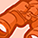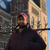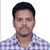New to Qlik Sense

If you’re new to Qlik Sense, start with this Discussion Board and get up-to-speed quickly.

Announcements
QlikWorld 2022, LIVE in Denver CO., May 16-19, 2022. REGISTER NOW TO RECEIVE EARLY BIRD PRICING
cancel
Showing results for
Did you mean:Contributor III

Aggr in calculated dimension

I have an aggr dimension that works:

aggr(if(rank(\$(vKPI_Global_Score_YTD))=2,[Customer]),[Customer])

It returns the Customer who has the 2nd highest score for the variable.

Now I want a calculated dimension that should work something like:

IF([Customer] = aggr(if(rank(\$(vKPI_Global_Score_YTD))=2,[Customer]),[Customer]) AND Zone = 'ABC', [Customer Zone])

I also tried something like:

aggr(if(Customer=aggr(if(rank(\$(vKPI_Global_Score_YTD))=2,[Customer]),[Customer]) and Zone ='ABC', [Customer Zone], [Customer Zone])

This however isn't working for both.

Any Idea?

9 RepliesPartner

maybe this:

=Only( {< Customer = {"\$(=rank(\$(vKPI_Global_Score_YTD)))=2"} >} [Customer Zone] )Champion

his second condition is missing:

=Only( {< Customer = {"\$(=rank(\$(vKPI_Global_Score_YTD)))=2"},Zone={'ABC'}>} [Customer Zone] )Specialist II

Hi

may be this

=Only( {< Customer = {"\$(=rank(\$(vKPI_Global_Score_YTD)))=2"},Zone ={'ABC'} >} [Customer Zone] )

or this

aggr(

if(

rank(\$(vKPI_Global_Score_YTD))=2 AND Zone ='ABC' ,[Customer]),[Customer])Creator II

May I know what is the expression stored in "vKPI_Global_Score_YTD".Creator II

aggr(if(rank(\$(vKPI_Global_Score_YTD))=2,[Customer]),[Customer])Contributor III
Author

Unfortunately your answers didn't workOnly results in a measure, I need it to result in a dimension.Contributor III
Author

It is a very complicated total score within +10 other variable calculations into it. The problem is that we need the score for just two dimensions without filtering. There is also no set analysis extra possible within the total score, so we are looking for a workaround.Partner

what's the chart object are you using to display the "Customer Zone" calculated dimension ?Contributor III
Author

So I have \$(vKPI_Global_YTD) as a measure to be display in Simple KPI extension. The idea is to limit the score by only a combination of two dimension values Customer and Zone, therefor I concatenate it, because you can only have one dimension in Simple KPI.

aggr(if(rank(\$(vKPI_Global_Score_YTD))=2,[Customer]),[Customer]) as a dimension, gives me the required result, but it also needs to be limited by zone='EUR'...

If I make a regular table with:

Kolom 1

aggr(if(rank(\$(vKPI_Global_Score_YTD))=2,[Customer]),[Customer])

Kolom 2

aggr(if(Zone='EUR',[Zone]),[Zone])

Kolom 3

\$(vKPI_Global_YTD)

I do get the required value in Kolom 3...Tags
Community Browser